# Learn Reinforcement Learning (4) - Actor-Critic, A2C, A3C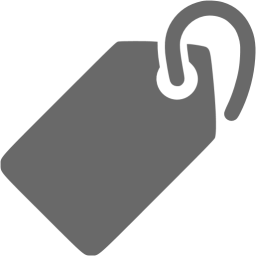In the previous article, we looked at several methods to improve the performance of the DQN algorithm and the Deep SARSA algorithm to solve the ball-find-3 problem in Grid World. Today we will look at Actor-Critic algorithms that outperform previous algorithms in ball-find-3, and visualize agent actions. We will also look at the A3C, which has further developed this algorithm.

## Actor-Critic Algorithm and A2C

The Dueling DQN we looked at last time was the idea of ​​dividing the network results into V and A before reassembling them before we got the Q value. Similar, but different Actor-Critic algorithm1 use two networks: an Actor network and a Critic network.

The Actor determines the action when the state is given, and Critic evaluates the value of the state.2 Dueling DQN is quite similar to Dueling DQN, but the Dueling DQN is the sum of the two values to get the Q value and the Actor-Critic does not combine the values at the end. Depending on the implementation, the decoders of the receiving network may join together, but the structure of the Dueling DQN and the Actor-Critic will differ depending on whether the Q values are combined or not. Of course, there is a difference between using DQN in Replay Buffer and not using Actor-Critic.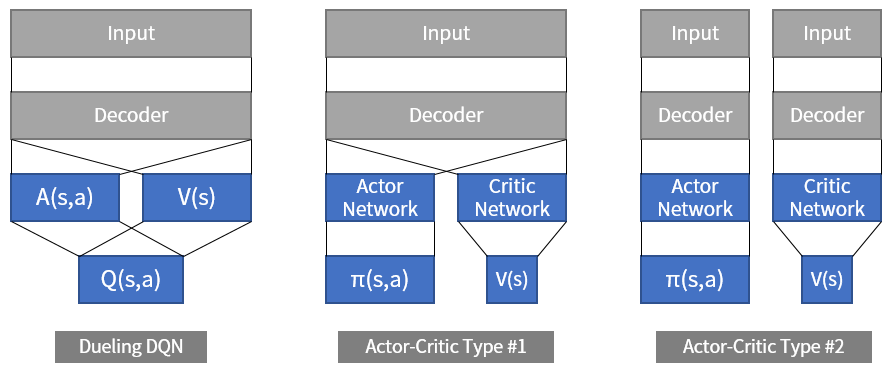Figure 1. The structure of the actor-critic algorithm can be divided into two types depending on whether or not parameter sharing is applied to the decoders that interpret the input.

The biggest difference between DQN and Actor-Critic that we have seen in the last article is whether to use Replay Buffer.3 Unlike DQN, Actor-Critic does not use Replay Buffer but learns the model using state(s), action(a), reward(r), and next state(s’) obtained at every step.

DQN obtains the value of $$Q(s,a)$$ and Actor-Critic obtains the value of $$\pi(s,a)$$ and $$V(s)$$. $$V(s)$$ is the value function we’ve covered so far, and $$\pi(s,a)$$ is the probability of taking a specific action in some state. Usually this probability can be obtained using softmax. Softmax calculates certain values by exponentiation with $$e$$ as the base, then sums and divides to convert the sum to a probability of 1.0.

$P(z) = \frac{e^{z_j}}{\sum^K_{k=1} e^{z_k}} \phantom{2} (for \phantom{1} j = 1, ... ,K)$

For example, if we have a value of [2,1,0] and convert it to softmax,

$sum = \sum^K_{k=1} e^{z_k} = e^2 + e^1 + e^0 = 11.1073$ $softmax = \frac{e^{z_j}}{\sum^K_{k=1} e^{z_k}} = \left[\frac{e^2}{sum}, \frac{e^1}{sum}, \frac{e^0}{sum}\right] = [0.67, 0.24, 0.09]$

It is a probability value of [0.67, 0.24, 0.09]. Softmax function is used in many areas of deep learning, such as image classification or text generation. Reinforcement learning can also be used to obtain the action probability of an agent.

The method of directly learning the behavior probability of an agent is called REINFORCE or policy gradient4. A policy is a policy about what action the agent will take, and a gradient means that the policy value is updated through differentiation and the optimal policy is searched. However, since the method of directly learning the action probability of the agent is unstable, it is the core of the Actor-Critic to increase the stability by using the value function together.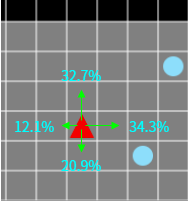Figure 2. The policy value directly represents the probability that each action(a) will occur in any state(s).

Using the Advantage as the expected output of Actor-Critic’s Actor will be Advantage Actor-Critic, A2C. Advantage is a value that determines how much better than expected ($$V(s)$$). Advantage generally uses the value obtained by subtracting $$V(s)$$ from $$Q(s,a)$$.

$A(s,a) = Q(s,a) - V(s)$

However,$$Q(s,a)$$ is not shown in the structure of the Actor-Critic algorithm in Figure 1. But as we discussed in the second article in this series,

$Actual Value \simeq Present Value + Future Value$

The present value is reward, and the future value is replaced with the value function $$V(s')$$ of the next state, the following expression can be obtained.

$Q(s,a) \simeq R + \gamma V(s')$

In summary, the formula for obtaining Advantage changes as follows.

$A(s,a) \simeq R + \gamma V(s') - V(s)$

The $$V(s)$$ value calculated by the Critic Network will also affect the calculation of the Actor Network.

The most famous example affected by the Actor-Critic algorithm is the Deep Mind’s AlphaGo. They learned the policy network corresponding to the actor and the value network corresponding to the critic so that they could find the optimal action in the given environment.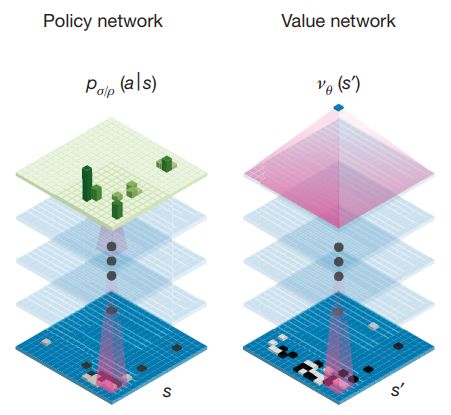Figure 3. Deep Mind’s AlphaGo learned agents through two networks: Policy Network and Value Network.

We can not create an AlphaGo at once, but we can train an agent with greatly improved action with the Actor-Critic algorithm in Grid World.

From about 100 episodes, the agent starts to look for the ball. Even if there is no ball in sight, the agent gets closer to the ball through navigation, and if there are multiple balls in sight, it almost reaches all the balls without missing.

How do these agents move? In Unity, we will look at ideas from Arthur Juliani’s blog article on ML-Agent, a reinforcement learning agent, to see how A2C agents judge the environment.

## Action visualization of A2C

Let’s run the agent by bringing up the learned network for 38,000 episodes with the A2C algorithm in ball-find-3. Load Model (Actor-Critic) button to load pre-learned models and weights stored on the web, and then to run them by pressing the Run (Actor-Critic) button.

The agent displays the probability of each action calculated by the Actor of the A2C network in green, and the detailed probability information is displayed in the cyan color at the bottom. The Run button changes to the PAUSE button at run time, so we can pause during execution and see how the current agent interprets the environment.

We can check how well each agent behaves when the agent is close to and far from the ball, when two or more balls come in at once into the field of view, or when there is nothing in the field of view.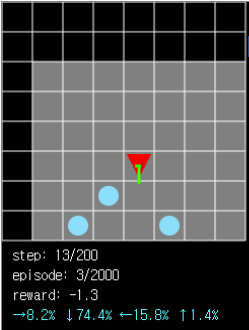Figure 4. When multiple balls are in view, the agent predicts the best action with the highest probability(down, 74.4%).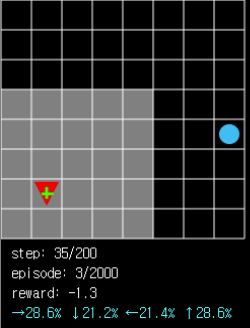Figure 5. When there is nothing in sight, the probability of moving in each direction is relatively even. However, you can see that there is a higher probability of moving to the right and up with less clogs (right, 28.6%> left, 21.4%).Figure 6. There is only 34.4% chance that the agent will move towards the ball even if the ball is in front of the one square. There is still room for improvement.

There is only 34.4% chance that the agent will move towards the ball even if the ball is in front of the one square. There is still room for improvement.

As shown in Figure 6, there is still a shortage of agents learned with the A2C algorithm. Especially because DQN does not utilize replay buffer but uses search data for learning immediately, it can result in bad results when learning wrong way. A3C, an algorithm for improving these disadvantages, was announced by the DeepMind in 2016.

## A3C

A3C stands for Asynchronous Advantage Actor-Critic. Asynchronous means running multiple agents instead of one, updating the shared network periodically and asynchronously. Agents update independently of the execution of other agents when they want to update their shared network.Figure 7. Structure of A3C shown in the figure. Each agent searches in a separate environment and exchanges learning results with the global network. Source Link

As we can see in Figure 7, A3C is a structure that executes multiple A2C agents independently and exchanges learning results with the global network. The advantage of running multiple agents in this way is that agent can learn from various data that can be obtained from various environments. The DQN we saw in the previous article also had the advantage of using a variety of experience because it uses replay buffer, but it also had the disadvantage of using old data. On the other hand, A3C does not have the disadvantage of DQN because it always learns using the latest data.

The A3C with four agents can be learned by pressing the Learn (A3C) button. Since agents move at the same time, the speed of agent movement is slower than usual. The learning ends when the total episode progression of each agent reaches 2000.

After learning the A3C network in this way, the average reward is shown graphically, and the A3C performs better than the other algorithms in the ball-find-3 problem.

In the initial learning, the reward of the A3C increases almost constantly, and as the learning continues, the performance differs from the A2C. Compared with the original DQN or the softupdate version of DQN, it is an improvement.

## Action visualization of A3C

So now we will visualize the actions of the A3C agent, which is smarter than the A2C, and see how well it moves compared to the A2C. In order to learn the best agents, agent learned A3C using 16 agents in 50,000 episodes in local environment.

As with the A2C example above, pressing the Load Model (A3C) button loads pre-learned models and weights stored on the Web and can be executed by pressing the Run (A3C) button.

The average reward is -1.0 to 0.0. You can see that the agent moves more precisely towards the goal.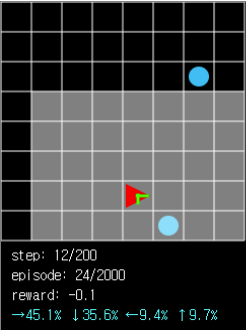Figure 8. When the ball is in close proximity, the probability of moving away from the ball has been reduced to one digit.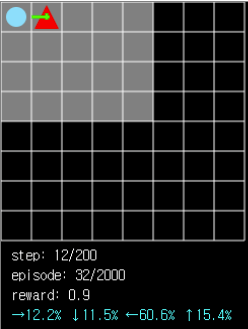Figure 9. In a situation similar to that of Figure 6, the probability of moving to ball has nearly doubled to 60%.

If we want to improve performance for a bit more than that, we can add memory to the agent. In other words, the state that agent saw in the past is memorized by LSTM and used to determine the current action. However, this example is too heavy to run on the web, so I will not implement it at this time.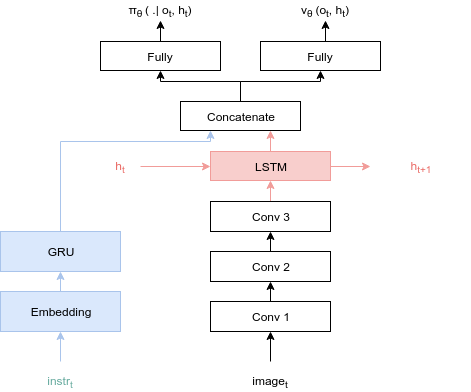Figure 10. It is the layer structure of the agent that solves problems similar to Grid World in the body. LSTM allows you to add memory to the agent. Source Link

A3C is a simple idea of using multiple agents instead of a single agent, but it has done a good job of being a baseline in performance. The latest algorithms that are similar to or better than A3C include DDPG, TRPO, PPO, TD3, and SAC. The details are listed in OpenAI’s reinforcement learning learning & organization site, Spinning Up.

Unlike the previous article, I hope you are more comfortable with visualization than formula. I have been solving one problem of ball-find-3 for a long time and I am trying to solve other problems, so I try to get more interesting problems next time. So this is the end of this post. Thank you for reading the long article.

1. The first Actor-Critic algorithm was used to solve the cart-pole problem in Neuron-like elements that can solve difficult learning control problems, a paper published in 1983 by A.Barto and R.Sutton.

2. In addition to using two networks in deep running, there are typically GAN(Generative Adversarial Networks). There is also Style Transfer, which combines two inputs(not two networks) to create a new output.

3. There is also an algorithm called ACER(Actor Critic with Experience Replay) that uses Replay Buffer in Actor-Critic. This was also announced in Deep Mind.